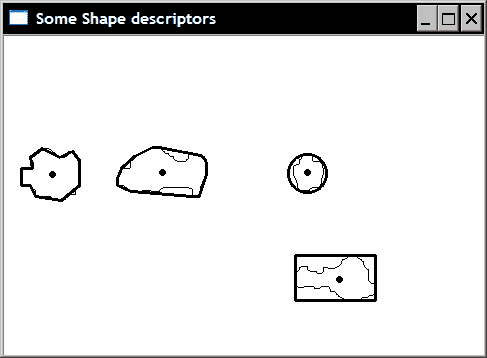# 七、提取直线，轮廓和零件

• 使用 Canny 运算符检测图像轮廓
• 使用霍夫变换检测图像中的直线
• 将线拟合到一组点
• 提取组件的轮廓
• 计算组件的形状描述符

# 使用 Canny 运算符检测图像轮廓

## 操作步骤

Canny 算法在 OpenCV 中通过函数cv::Canny实现。 如将要解释的，该算法需要指定两个阈值。 因此，对该函数的调用如下：

   // Apply Canny algorithm
cv::Mat contours;
cv::Canny(image,    // gray-level image
contours, // output contours
125,      // low threshold
350);     // high threshold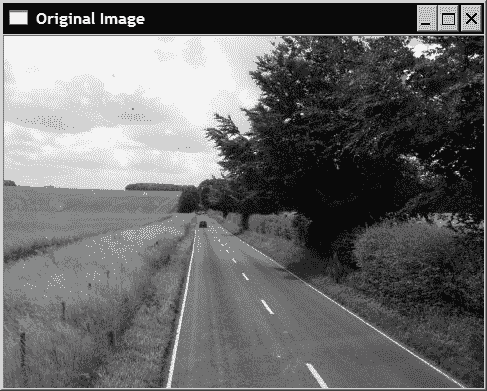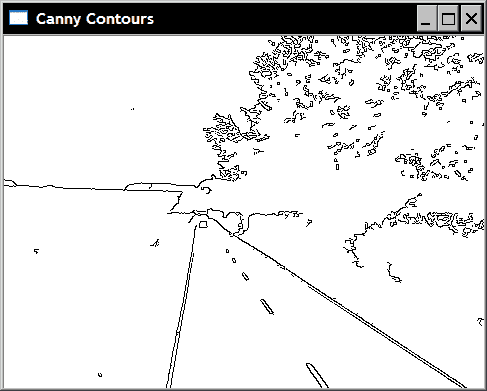cv::Mat contoursInv; // inverted image
cv::threshold(contours,contoursInv,
128,   // values below this
255,   // becomes this
cv::THRESH_BINARY_INV);


## 工作原理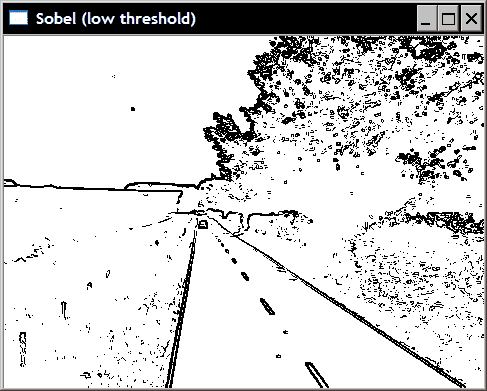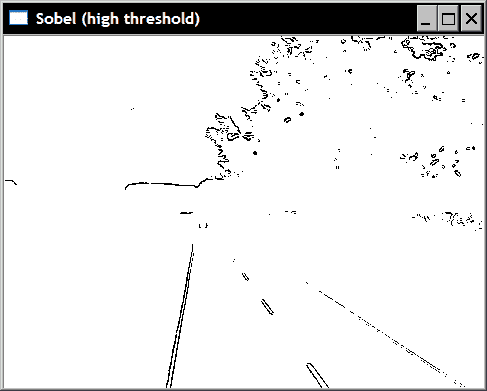## 另见

The classic article by J. Canny, A computational approach to edge detection, IEEE Transactions on Pattern Analysis and Image Understanding, vol. 18, issue 6, 1986.


# 使用霍夫变换检测图像中的直线

## 准备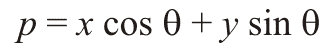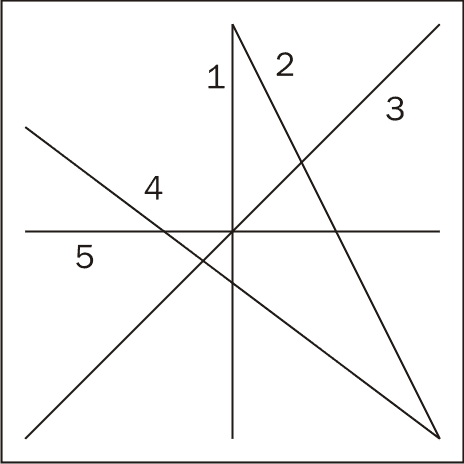## 操作步骤

OpenCV 为行检测提供了霍夫变换的两种实现。 基本版本是cv::HoughLines。 它的输入是一个二进制映射，其中包含一组点（用非零像素表示），其中一些点对齐形成线。 通常，它是例如从 Canny 运算符获得的边缘图。 cv::HoughLines函数的输出是cv::Vec2f元素的向量，每个元素都是一对浮点值，它们代表检测到的线的参数(ρ, θ)。 这是使用此函数的示例，其中我们首先应用 Canny 运算符来获取图像轮廓，然后使用霍夫变换检测线：

   // Apply Canny algorithm
cv::Mat contours;
cv::Canny(image,contours,125,350);
// Hough tranform for line detection
std::vector<cv::Vec2f> lines;
cv::HoughLines(test,lines,
1,PI/180,  // step size
80);       // minimum number of votes


   std::vector<cv::Vec2f>::const_iterator it= lines.begin();
while (it!=lines.end()) {

float rho= (*it);   // first element is distance rho
float theta= (*it); // second element is angle theta

if (theta < PI/4\.
|| theta > 3.*PI/4.) { // ~vertical line

// point of intersection of the line with first row
cv::Point pt1(rho/cos(theta),0);
// point of intersection of the line with last row
cv::Point pt2((rho-result.rows*sin(theta))/
cos(theta),result.rows);
// draw a white line
cv::line( image, pt1, pt2, cv::Scalar(255), 1);

} else { // ~horizontal line

// point of intersection of the
// line with first column
cv::Point pt1(0,rho/sin(theta));
// point of intersection of the line with last column
cv::Point pt2(result.cols,
(rho-result.cols*cos(theta))/sin(theta));
// draw a white line
cv::line(image, pt1, pt2, cv::Scalar(255), 1);
}

++it;
}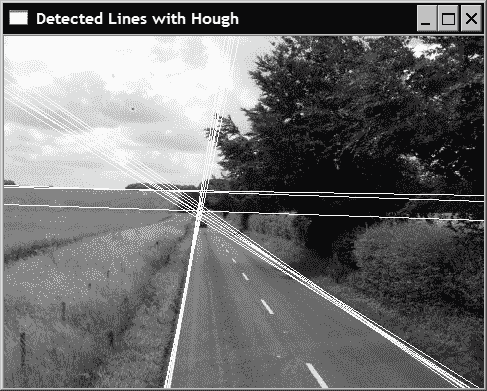class LineFinder {

private:

// original image
cv::Mat img;

// vector containing the end points
// of the detected lines
std::vector<cv::Vec4i> lines;

// accumulator resolution parameters
double deltaRho;
double deltaTheta;

// minimum number of votes that a line
// must receive before being considered
int minVote;

// min length for a line
double minLength;

// max allowed gap along the line
double maxGap;

public:

// Default accumulator resolution is 1 pixel by 1 degree
// no gap, no mimimum length
LineFinder() : deltaRho(1), deltaTheta(PI/180),
minVote(10), minLength(0.), maxGap(0.) {}


     // Set the resolution of the accumulator
void setAccResolution(double dRho, double dTheta) {

deltaRho= dRho;
deltaTheta= dTheta;
}

// Set the minimum number of votes
void setMinVote(int minv) {

minVote= minv;
}

// Set line length and gap
void setLineLengthAndGap(double length, double gap) {

minLength= length;
maxGap= gap;
}


     // Apply probabilistic Hough Transform
std::vector<cv::Vec4i> findLines(cv::Mat& binary) {

lines.clear();
cv::HoughLinesP(binary,lines,
deltaRho, deltaTheta, minVote,
minLength, maxGap);

return lines;
}


     // Draw the detected lines on an image
void drawDetectedLines(cv::Mat &image,
cv::Scalar color=cv::Scalar(255,255,255)) {

// Draw the lines
std::vector<cv::Vec4i>::const_iterator it2=
lines.begin();

while (it2!=lines.end()) {

cv::Point pt1((*it2),(*it2));
cv::Point pt2((*it2),(*it2));

cv::line( image, pt1, pt2, color);

++it2;
}
}


   // Create LineFinder instance
LineFinder finder;

// Set probabilistic Hough parameters
finder.setLineLengthAndGap(100,20);
finder.setMinVote(80);

// Detect lines and draw them
std::vector<cv::Vec4i> lines= finder.findLines(contours);
finder.drawDetectedLines(image);
cv::namedWindow("Detected Lines with HoughP");
cv::imshow("Detected Lines with HoughP",image);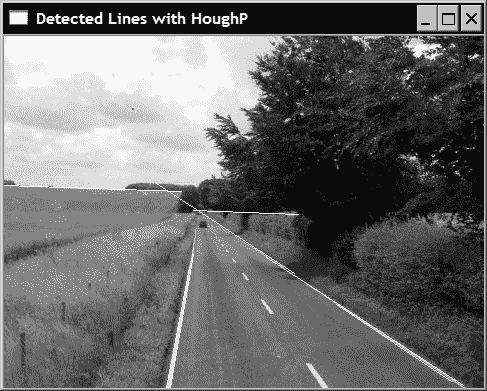## 工作原理

   // Create a Hough accumulator
// here a uchar image; in practice should be ints
cv::Mat acc(200,180,CV_8U,cv::Scalar(0));


   // Choose a point
int x=50, y=30;

// loop over all angles
for (int i=0; i<180; i++) {

double theta= i*PI/180.;

// find corresponding rho value
double rho= x*cos(theta)+y*sin(theta);
// j corresponds to rho from -100 to 100
int j= static_cast<int>(rho+100.5);

std::cout << i << "," << j << std::endl;

// increment accumulator
acc.at<uchar>(j,i)++;
}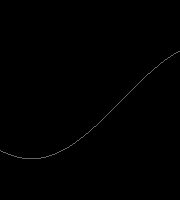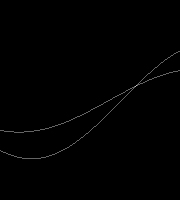cv::HoughLines(test,lines,1,PI/180,60);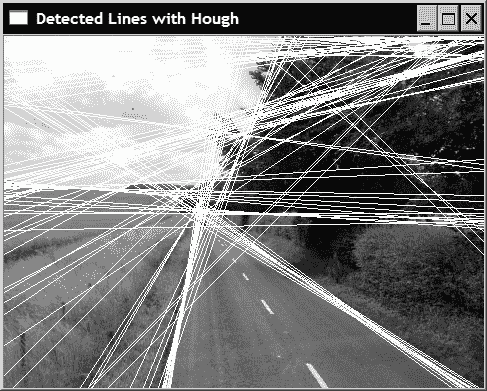## 更多

### 检测圆形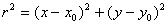cv::GaussianBlur(image,image,cv::Size(5,5),1.5);
std::vector<cv::Vec3f> circles;
2,   // accumulator resolution (size of the image / 2)
50,  // minimum distance between two circles
200, // Canny high threshold
100, // minimum number of votes
25, 100); // min and max radius


   std::vector<cv::Vec3f>::
const_iterator itc= circles.begin();

while (itc!=circles.end()) {

cv::circle(image,
cv::Point((*itc), (*itc)), // circle centre
cv::Scalar(255), // color
2);              // thickness

++itc;
}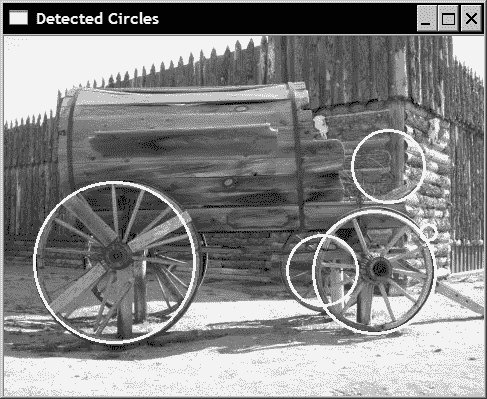### 广义霍夫变换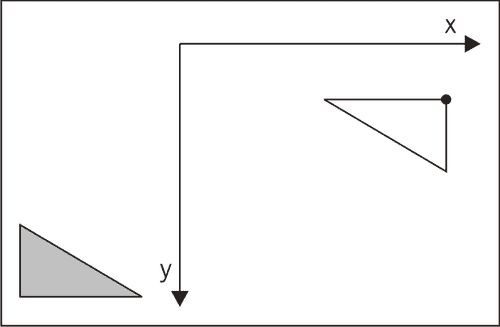## 另见

The article Gradient-based Progressive Probabilistic Hough Transform by C. Galambos, J. Kittler, and J. Matas, IEE Vision Image and Signal Processing, vol. 148 no 3, pp. 158-165, 2002. It is one of the numerous reference on the Hough transform and describes the probabilistic algorithm implemented in OpenCV.

The article by H.K. Yuen, J. Princen, J. Illingworth, and J Kittler, Comparative Study of Hough Transform Methods for Circle Finding, Image and Vision Computing, vol. 8 no 1, pp. 71-77, 1990 that describes different strategies for circle detection using the Hough transform.


# 将直线拟合到一组点

## 操作步骤

   int n=0; // we select line 0
// black image
cv::Mat oneline(contours.size(),CV_8U,cv::Scalar(0));
// white line
cv::line(oneline,
cv::Point(lines[n],lines[n]),
cv::Point(lines[n],lines[n]),
cv::Scalar(255),
5);
// contours AND white line
cv::bitwise_and(contours,oneline,oneline);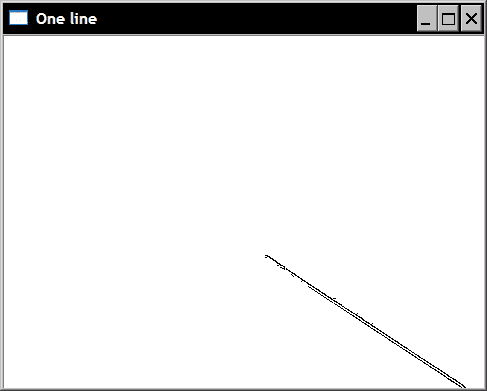std::vector<cv::Point> points;

// Iterate over the pixels to obtain all point positions
for( int y = 0; y < oneline.rows; y++ ) {
// row y

uchar* rowPtr = oneline.ptr<uchar>(y);

for( int x = 0; x < oneline.cols; x++ ) {
// column x

// if on a contour
if (rowPtr[x]) {

points.push_back(cv::Point(x,y));
}
}
}


   cv::Vec4f line;
cv::fitLine(cv::Mat(points),line,
CV_DIST_L2, // distance type
0,          // not used with L2 distance
0.01,0.01); // accuracy


   int x0= line;        // a point on the line
int y0= line;
int x1= x0-200*line; // add a vector of length 200
int y1= y0-200*line; // using the unit vector
cv::line(image,cv::Point(x0,y0),cv::Point(x1,y1),
cv::Scalar(0),3);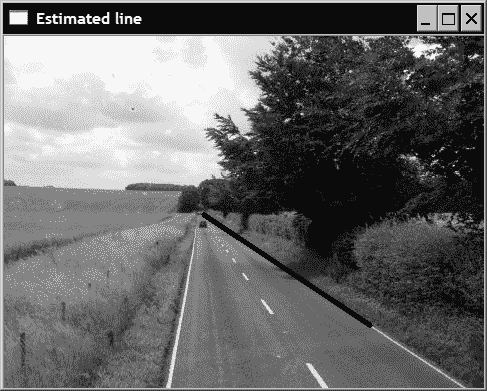## 更多

   cv::RotatedRect rrect= cv::fitEllipse(cv::Mat(points));
cv::ellipse(image,rrect,cv::Scalar(0));


# 提取组件的轮廓## 操作步骤

OpenCV 提供了一个简单的函数，可以提取图像的已连接组件的轮廓。 它是cv::findContours函数：

   std::vector<std::vector<cv::Point>> contours;
cv::findContours(image,
contours, // a vector of contours
CV_RETR_EXTERNAL, // retrieve the external contours
CV_CHAIN_APPROX_NONE); // all pixels of each contours


   // Draw black contours on a white image
cv::Mat result(image.size(),CV_8U,cv::Scalar(255));
cv::drawContours(result,contours,
-1, // draw all contours
cv::Scalar(0), // in black
2); // with a thickness of 2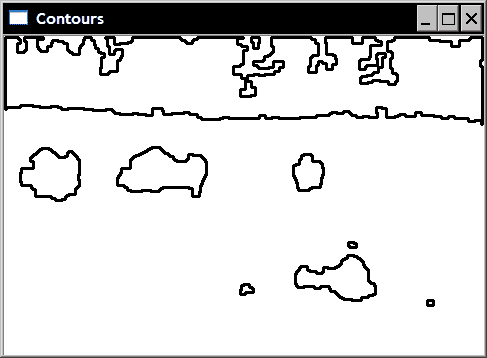## 工作原理

   // Eliminate too short or too long contours
int cmin= 100;  // minimum contour length
int cmax= 1000; // maximum contour length
std::vector<std::vector<cv::Point>>::
const_iterator itc= contours.begin();
while (itc!=contours.end()) {

if (itc->size() < cmin || itc->size() > cmax)
itc= contours.erase(itc);
else
++itc;
}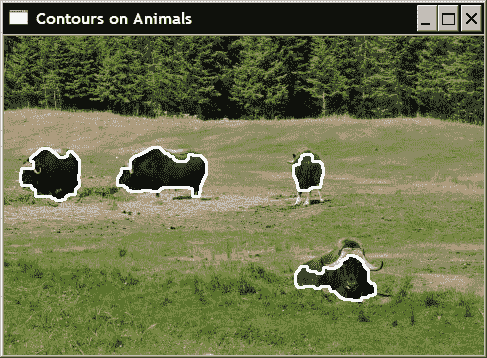## 更多

   cv::findContours(image,
contours, // a vector of contours
CV_RETR_LIST, // retrieve all contours
CV_CHAIN_APPROX_NONE); // all pixels of each contours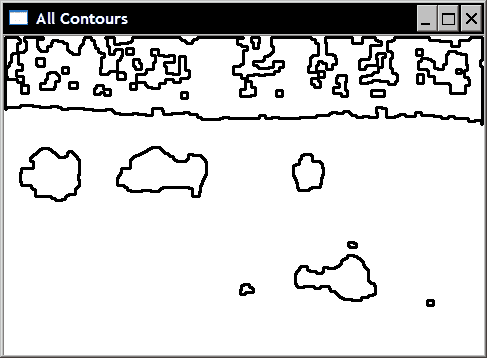std::vector<cv::Vec4i> hierarchy;
cv::findContours(image,
contours, // a vector of contours
hierarchy, // hierarchical representation
CV_RETR_TREE, // retrieve all contours in tree format
CV_CHAIN_APPROX_NONE); // all pixels of each contours


# 计算组件的形状描述符

## 操作步骤

   // testing the bounding box
cv::Rect r0= cv::boundingRect(cv::Mat(contours));
cv::rectangle(result,r0,cv::Scalar(0),2);


   // testing the enclosing circle
cv::Point2f center;
cv::circle(result,cv::Point(center),


   // testing the approximate polygon
std::vector<cv::Point> poly;
cv::approxPolyDP(cv::Mat(contours),poly,
5,     // accuracy of the approximation
true); // yes it is a closed shape


   // Iterate over each segment and draw it
std::vector<cv::Point>::const_iterator itp= poly.begin();
while (itp!=(poly.end()-1)) {
cv::line(result,*itp,*(itp+1),cv::Scalar(0),2);
++itp;
}
// last point linked to first point
cv::line(result,
*(poly.begin()),
*(poly.end()-1),cv::Scalar(20),2);


   // testing the convex hull
std::vector<cv::Point> hull;
cv::convexHull(cv::Mat(contours),hull);


   // testing the moments

// iterate over all contours
itc= contours.begin();
while (itc!=contours.end()) {

// compute all moments
cv::Moments mom= cv::moments(cv::Mat(*itc++));

// draw mass center
cv::circle(result,
// position of mass center converted to integer
cv::Point(mom.m10/mom.m00,mom.m01/mom.m00),
2,cv::Scalar(0),2); // draw black dot
}